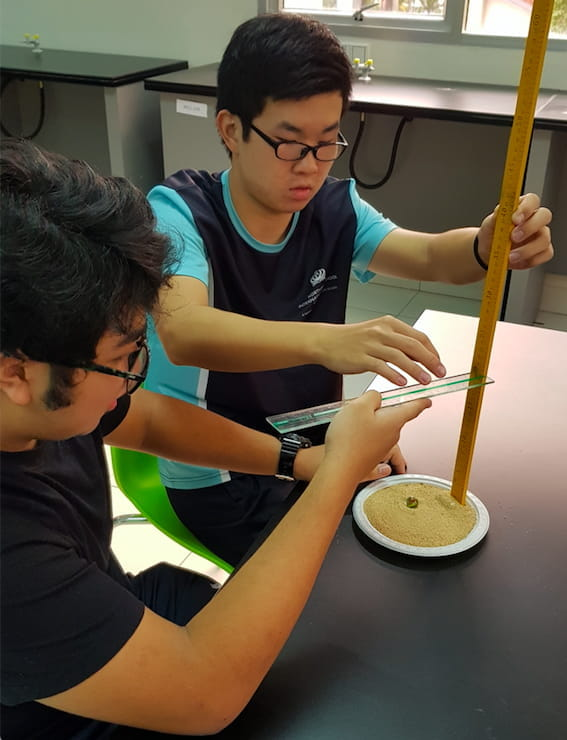WRITTEN BY
Nord Anglia
29 January, 2020

# To learn maths at Northbridge, a child must first develop their visual memory and perceptionTo learn maths at Northbridge, a child must first develop their visual memory and perception Visual perception is the brain’s ability to understand what the eyes see. Visual memory is being able to remember what has been seen. They are extremely important skills that every child at Northbridge International School Cambodia must develop in order to be a successful mathematician.

Visual perception is the brain’s ability to understand what the eyes see. Visual memory is being able to remember what has been seen. They are extremely important skills that every child at Northbridge International School Cambodia must develop in order to be a successful mathematician.

Above; a skyscraper puzzle.

Visual perception and visual memory are used to: recognise mathematical symbols; + - x ÷, develop a good sense of direction; north, south, east, west, recognise sets of numbers, order amounts, compare shapes/sizes/distances, draw symmetrical patterns, draw shapes, give and follow directions and many other skills used in maths and in everyday situations.

On Monday, teachers learnt about different ways to help our students to develop their visual perception and memory. Some of the ways are exploring visual patterns, solving puzzles, talking about similarities and differences between pictures and sets and exploring manipulatives.

Manipulatives are physical objects that are used to develop an understanding of mathematical concepts and are an important part of teaching and learning mathematics for learners of every age.

The following pictures show some of the patterns and puzzles that teachers explored on Monday.

Above; staircase patterns showing 6x6 and 10x10.Above; a pattern showing square numbers.

Using manipulatives creates the opportunity for children to talk about their learning. Talking about maths allows children to develop their understanding of how numbers relate to one another. Having a secure understanding of what numbers actually mean, enables children to develop a range of skills for calculating.

For example: understanding that 7 is 5 + 2 means that 15 + 7 can be much easier to solve; you can then do 15 + 5 = 20 then add 2 which is 22. Or for a more challenging question; understanding that 999 is 1000 - 1 means you can answer 7 x 999 by first doing 7 x 1000 and then subtracting 7 x 1 which is 6993.

Talking about maths also helps children to develop their understanding through convincing and reasoning, having to convince a friend that their method works shows how well a child understands what is happening in the calculation.Above; a staircase pattern and pattern that equals 100.

Above: Mr Mike and Ms Laurie found out the value of their names using Cuisenaire rods. Mike = 48 and Laurie = 78.